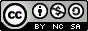# 线性回归

## 基于大脑体积预测大脑重量

### 输入特征

• 大脑体积：以立方厘米为单位

### 预测结果## 房价预测

### 输入特征

• CRIM：房子所在街区的犯罪率
• ZN：划分为大于 25,000 平方英尺的住宅用地的比例。
• INDUS：房子所在街区的非零售营业面积占比
• CHAS：Charles River虚变量，为1表示tract bounds river
• NOX：一氧化氮浓度（百万分之一）
• RM：每个住宅的平均房间数
• AGE：1940 年之前建造的自住单元的比例
• DIS：到五个波士顿就业中心的加权距离
• TAX：每 10,000 美元的全值财产税率
• PTRATIO：按城镇划分的师生比例
• B：1000(Bk — 0.63)²，其中 Bk 是按城镇划分的非裔美国人后裔的比例
• LSTAT：人口较低地位的百分比

### 预测结果## 模型解释

$X$是特征输入，其形状为[BatchSize, InputSize]；$Y$是对应特征的输出，其形状为[BatchSize, OutputSize]

• $W$形状为[InputSize, OutputSize]
• $B$形状为[OutputSize]

$$Y' = X \cdot W + B$$

$$D = Y' - Y$$

$$L = mean(D^2)$$

$$W = W - \alpha \cdot gradW$$

$$B = B - \alpha \cdot gradB$$

# 逻辑回归

## 糖尿病预测

### 输入特征

• Pregnancies：孕期，以周为单位
• Glucose：血糖水平，以mg/dL为单位
• BloodPressure：血压，以mmHg为单位
• SkinThickness：皮肤厚度，以毫米为单位
• Insulin：胰岛素水平，以单位（unit）衡量
• BMI：身高体重指数
• DiabetesPedigreeFunction：糖尿病遗传系数
• Age：年龄

#### 血糖数据分类可视化### 预测结果

• Accuracy：0.7208
• Precision：0.6970
• Recall：0.4107
• F1：0.5169

## 模型解释

$X$是特征输入，其形状为[BatchSize, InputSize]；$Y$是对应特征的输出，其形状为[BatchSize, OutputSize]，其中$y_i$的值应该是0或1，而不应当出现其他值。

• $W$形状为[InputSize, OutputSize]
• $B$形状为[OutputSize]

$$Y' = \sigma(X \cdot W + B)$$

$$\sigma(X) = \frac{1}{1+e^{-X}}$$

$$L = -\frac{1}{BatchSize}\sum_{i=1}^{OutputSize} Y_i \cdot logY'_i + (1 - Y_i)log(1-Y'_i)$$

$$W = W - \alpha \cdot gradW$$

$$B = B - \alpha \cdot gradB$$

# 神经网络

## 心脏病预测

### 输入特征

• age：年龄
• sex：性别

• 0：女性
• 1：男性
• cp：胸痛分类

• 1：典型心绞痛
• 2：非典型心绞痛
• 3：非心绞痛
• 4：无症状
• trestbps：舒张压，以mmHg为单位
• chol：血清胆固醇水平，以mg/dL为单位
• fbs：空腹血糖

• 1：空腹血糖高于120mg/dL
• 0：空腹血糖低于或等于120mg/dL
• restecg：静息状态心电图结果：

• 0：正常
• 1：ST-T波异常（T波倒置和/或ST段偏差大于0.05毫伏）
• 2：根据Estes标准表明可能或明确的左心室肥厚
• thalach：最大心率
• exang：运动诱发心绞痛

• 1：有
• 0：无
• oldpeak：运动引起的ST电压降低

• 1：有
• 0：无
• slope：峰值运动ST段斜率

• 1：升高
• 2：平坦
• 3：下降
• ca：荧光检查着色的主要血管数（0~3）
• thal：地中海贫血

• 3：正常
• 6：固定缺陷
• 7：可逆缺陷

### 预测结果

Loss与迭代关系图：• Accuracy：0.7869
• Precision：0.7941
• Recall：0.8182
• F1 Score：0.8060

## 模型解释$$Y_i' = \sigma(Y_{i-1}'\cdot W_i + B_i)$$

# 卷积神经网络

## 新冠肺炎CT成像预测

### 输入特征### 预测结果• covid：患有新冠肺炎
• normal：健康
• viral：其他病毒引起的肺炎

• Accuracy：0.9111
• Precision：0.9139
• Recall：0.9111
• F1 Score：0.9102

## 模型解释

LeNet结构如下：LeNet的主要特征是以一层卷积和一层池化为一组，连续两组，随后转全连接层进行多分类。

ReLU：

$$ReLU(x) = max(0, x)$$

$$\sigma(\mathbf{z})_i = \frac{e^{z_i}}{\sum_{i=1}^{OutputSize} e^{z_i}}$$

$$L = - logY_i$$

# 循环神经网络

## 新冠肺炎确诊人数趋势预测

### 输入特征

• 前一天的统计人数（2020年1月22日~同年7月11日）

### 预测结果## 模型解释

$$h^{(t)} = \sigma_1(Ux^{(t)} + Wh^{(t-1)} + b)$$

$$y'^{(t)} = \sigma_2(o^{(t)}) = \sigma_2(Vh^{(t)} + c)$$LSTM神经元除了上面的$h^{(t)}$外，每时刻还存在一个细胞状态矩阵$C^{(t)}$，同时还有遗忘门、输入门和输出门。

$$f^{(t)} = \sigma(W_fh^{(t-1)} + U_fx^{(t)} + b_f)$$

$$i^{(t)} = \sigma(W_ih^{(t-1)} + U_ix^{(t)} + b_i)$$

$$a^{(t)} = tanh(W_ah^{(t-1)} + U_ax^{(t)} + b_a)$$

$$C^{(t)} = C^{(t-1)}\odot f^{(t)} + i^{(t)} \odot a^{(t)}$$

$$o^{(t)} = \sigma(W_oh^{(t-1)} + U_ox^{(t)} + b_o)$$

$$h^{(t)} = o^{(t)}\odot tanh(C^{(t)})$$

# 深度学习网络模型部署

## 系统前端输入## 系统结果输出• 患有新冠肺炎
• 没有感染任何肺炎（健康）
• 患有病毒引起的肺炎

## 后记

// Basic env
implementation "org.jetbrains.kotlin:kotlin-stdlib-jdk8:1.5.20"
implementation "ch.qos.logback:logback-classic:1.2.3"

// Utils
implementation group: 'com.opencsv', name: 'opencsv', version: '5.5'
implementation 'org.knowm.xchart:xchart:3.8.0'

// Javalin and template
implementation 'io.javalin:javalin:3.13.9'
implementation 'gg.jte:jte:1.10.0'

// Deeplearning4j
implementation 'org.deeplearning4j:deeplearning4j-core:1.0.0-beta7'
implementation 'org.deeplearning4j:deeplearning4j-ui:1.0.0-beta7'
implementation 'org.nd4j:nd4j-native:1.0.0-beta7'
implementation 'org.nd4j:nd4j-native:1.0.0-beta7:windows-x86_64-avx2'《医疗人工智能》课程报告天空 Blond 采用 知识共享 署名 - 非商业性使用 - 相同方式共享 4.0 国际 许可协议进行许可。